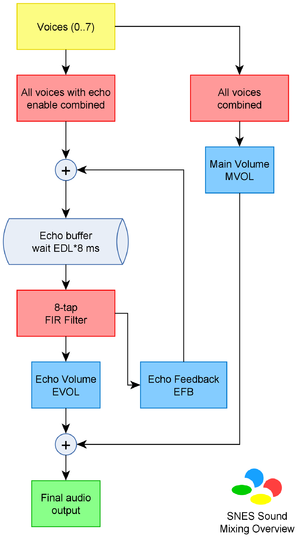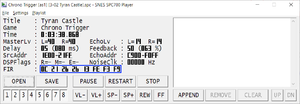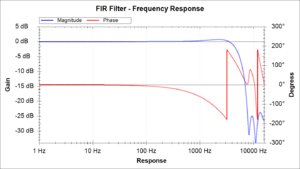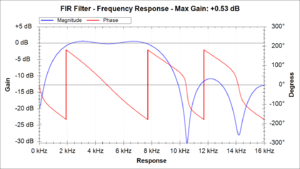# FIR FilterSNES sound mixing diagram. The FIR Filter plays an important role for the final sound output.

A FIR Filter (Finite impulse response filter) is a type of filter used on signal processing. It works by taking the sum of last Nth samples multiplied by a value, called FIR taps or coefficients. It's finite because if you pass a FIR filter in an impulse response, the impulse will fade out after passing though the N taps. That's easy to notice since the FIR filter never uses itself as feed unlike the IIR Filter.

On the SNES, the FIR filter has 8 taps which are 1.7 fixed point values. The filter is applied on the echo output so it has direct influence to the sound output and can be used to archive different effects which is more detailed on the following topics.

## Mathematical Definition

The FIR filter can be defined in the following mathematical formula: $y[n]=\sum _{i=0}^{N-1}b_{i}\cdot x[n-i]$For the current output sample Y[n], take the sum of previous N samples from source (including current), multiplied by the FIR coefficient, which is:

$y[n]=b_{0}\cdot x[n]+b_{1}\cdot x[n-1]+b_{2}\cdot x[n-2]+...+b_{N-1}\cdot x[n-(N-1)]$The SNES has eight FIR taps, which limits N to 8:

$y[n]=\sum _{i=0}^{7}b_{i}\cdot x[n-i]$However, the samples are processed from the oldest to the newest sample. That means the first FIR tap is applied to the oldest sample while the last FIR tap is applied to the newest sample:

$y[n]=\sum _{i=0}^{7}b_{i}\cdot x[n-(7-i)]$Which in other words, it yields to the equivalent pseudo-code:

y[n] = FIR * x[n - 7] +
FIR * x[n - 6] +
FIR * x[n - 5] +
FIR * x[n - 4] +
FIR * x[n - 3] +
FIR * x[n - 2] +
FIR * x[n - 1] +
FIR * x[n - 0];


Where FIR is a array containing the eight taps or coefficients.

## S-DSP ImplementationExample of FIR filter used on Chrono Trigger, which is used as an echo low-pass filter on this case. Screenshot taken from SNES SPC700 PLAYER

The FIR taps are located on the DSP registers $0F though$7F, where $0F is the first tap (FIR) and$7F is the last tap (FIR).

Because the S-DSP doesn't have floating point capability, the tap values are actually in the 1.7 fixed point, signed format. This means that values between $00-$7F is positive (0 to 127) and $80-$FF is negative (-128 to -1) and after doing the multiplication the value then is divided by 128. So for positive values, $7F means 1.00,$40 means 0.50, $20 means 0.25 and it goes on, while for negative values$80 means -1.00, $C0 means 0.50,$E0 means 0.25 and it goes on.

The coefficients sum is done on a 16-bit integer type, which means that if an overflow occur the value gets clipped. The only exception for this is the last multiplication (last FIR coefficient multiplied by the first sample). For this case, the number gets clamped instead of clipped, e.g.: 18623 + 16888 will yield to 32767 instead of overflowing to -30025.

An accurate implementation of the FIR filter given the above rules would be:

S = (FIR * x[n - 7] >> 6) +
(FIR * x[n - 6] >> 6) +
(FIR * x[n - 5] >> 6) +
(FIR * x[n - 4] >> 6) +
(FIR * x[n - 3] >> 6) +
(FIR * x[n - 2] >> 6) +
(FIR * x[n - 1] >> 6);


The ">>" operator is the arithmetic right shift operation, which on this context is the equivalent by dividing the value by 128 (without decimal places).

With the first 7 taps calculated, we clip it so it's always within the -32768 to 32767 range:

S = S & 0xFFFF;


Now we add the last tap which is multiplied by the current sample, x[n]:

S = S + (FIR * x[n] >> 6);


clamp it to the -32768 to 32767:

S = S > 32767 ? 32767 : S;
S = S < -32768 ? -32768 : S;


It's important to remember that the Echo buffer uses 15-bit samples instead of 16-bit and it's left-aligned, so the binary format is: "seee eeee eeee eee0" and not "ssee eeee eeee eeee". However, when the sample is placed on the FIR ring buffer (x[]), it end ups right shifted so it gets to the "ssee eeee eeee eeee" format.

So we finally take the last bit and we have our FIR value done:

Result = S & 0xFFFE;


Each sound channel (left and right) is processed separately.

Here is a diagram of the FIR filter calculation:

Because of the integer clipping behavior on the first seven taps, it's important to your FIR filter maximum gain never exceed 0 dB (or the absolute sum of all FIR taps never exceed 128) for avoiding audio clicks, specially with the first seven taps. Not all games obey that, so the most important is checking out the frequency response of the filter and verifying how the gain behaves for the frequency range.

Once done, the FIR is multiplied by the L/R echo volume and it's output together the main volume. It's also multiplied by the echo feedback value and fed back to the echo buffer together the sound output, as you can see on the above diagram.

### Timing Information

The S-DSP generates one stereo sample every 32 SPC700 clocks. During these 32 clocks, some operations are done by the S-DSP regarding echo and FIR filter. These are the following:

Each time a new sample is generated on the S-DSP, one sample is taken from the echo buffer and inserted on the FIR ring buffer (x[]), which then the ring buffer is used to generate the FIR sample output:

• The left echo channel is inserted on the ring buffer at cycle 22 and written on cycle 29.
• The right echo channel is inserted on the ring buffer at cycle 23 and written on cycle 30.

The FIR taps are not read immediately, but gradually read while the left and right echo channel are being processed:

• FIR is accessed during cycle 22.
• FIR and FIR are accessed during cycle 23.
• FIR, FIR and FIR are accessed during cycle 24.
• FIR and FIR are accessed during cycle 25.

## Frequency responseThe FIR Filter frequency response of one of the Chrono Trigger songs. Image generated via !vb fir command of VilelaBot. FIR used: $0C$21 $2B$2B $13$FE $F3$F9. It's a low-pass filter with a cut-off frequency of around ~4.5 kHz.

It's possible to view the frequency response of a FIR filter and therefore visualize which frequencies are amplified or attenuated. The calculations involves Discrete-time Fourier transform (DFFT) and are considerably complex. The formula looks like this:

$H(\jmath \omega )=\sum _{n=0}^{N-1}h(n)[cos(n\omega )-\jmath sin(n\omega )]$Where:

• $H(\jmath \omega )$is the output power for given frequency $H(\omega )$. It is a complex number, therefore you will end up having two components, which is the magnitude $|H(\jmath \omega )|$and phase $\measuredangle H(\jmath \omega )$.
• Magnitude is the output volume e.g. 1.0 is 100% volume.
• Phase means how late or early is the signal relative to the original, in radians ($\pi =+180^{\circ }$). Humans normally don't perceive phase changes, but combining two close and oppose phased-signals can generate some odd effects like an oscillating signal used on Square SPC engines.
• $h(n)$is the FIR filter taps.
• $N$is the amount of taps.

Disregarding SNES clipping and clamping effects, the frequency response of its 8-tap FIR would be calculated as the following:

$H(\jmath \omega )=\sum _{n=0}^{7}{\textrm {FIR}}(7-n)[cos(n\omega )-\jmath sin(n\omega )]$Expanding the sum yields:

$H(\jmath \omega )={\textrm {FIR}}(7)+{\textrm {FIR}}(6)[cos(\omega )-\jmath sin(\omega )]+{\textrm {FIR}}(5)[cos(2\omega )-\jmath sin(2\omega )]+{\textrm {FIR}}(4)[cos(3\omega )-\jmath sin(3\omega )]+{\textrm {FIR}}(3)[cos(4\omega )-\jmath sin(4\omega )]+{\textrm {FIR}}(2)[cos(5\omega )-\jmath sin(5\omega )]+{\textrm {FIR}}(1)[cos(6\omega )-\jmath sin(6\omega )]+{\textrm {FIR}}(0)[cos(7\omega )-\jmath sin(7\omega )]$When doing a visualization, it's often common to see ripples or signal ringing on certain regions. Even with careful values choice, it's not possible to attenuate them because of the low amount of taps the SNES FIR filter has, but regardless of the amount of taps they are often common on modern DSP applications and other kind of filters.

VilelaBot has a command for viewing FIR filters frequency response and gain, see the !vb help fir command for more information.

## Uses

The most useful thing that you can do with FIR filters is amplifying or attenuating a frequency range. Effectively, you can create your own sound equalizer depending on your echo settings and main volume combinations.

The most common usage involve:

• Using FIR as a low or high pass filters, normally using 4.5 kHz as the cut-off point:
• Low-pass to filter out high frequencies from the echo sound output.
• High-pass to filter out low frequencies from the echo sound output.
• Band-pass or band-stop filters to allow or disallow a frequency range:
• Band-pass allows frequency between A and B.
• Band-stop disallows frequency between A and B.
• Attenuate very specific frequencies that to balance the sound echo feedback and avoid overflows.
• Identity filter ($7F on the first tap and$00 on others): output original produced song from the echo buffer.

### Getting started

For who wants to start experiment with FIR filters, the best recommendation is start experimenting with filters already made and available under the examples section. Normally the most effects you want to archive is making your echo sound more filled and the filters that will (likely) do that, depending on your echo, are either the low-pass or high-pass filters. They will either let the lower frequencies (bass) pass or the higher frequencies (treble), balancing the amount of frequencies your song has.

Choose a filter that has a gain between 0.00 dB and -3.00 dB, which are safe filters that will not cause audio clipping/overflowing. Filters with positive gain are not recommended specially since during the internal FIR calculations some samples may end up overflowing and generating audio clicks. Another common problem is the combination of excessive Echo feedback that combined with FIR filter can make the echo buffer gradually get louder and eventually "exploding" your song.

Filters with low gain (-7.00 dB and below) will make your overall echo weakier and less notable, which will require you compensating it by increasing echo feedback and echo volume, but at the same time the song quality can slight reduce because a few bits will be lost during the calculation and won't be recovered since all calculations are fixed-point.

The Nintendo SPC driver (N-SPC) includes four standard FIR filters which can be used on your songs. These are:Analysis of N-SPC's built-in band-pass filter. It's notable that frequencies after 10 kHz has some ripples that still makes them slight audible.

1. $7F$00 $00$00 $00$00 $00$00

The identity filter. Used if you want to keep your echo sound the same as original, disregarding the echo feedback of course.

2. $58$BF $DB$F0 $FE$07 $0C$0C

High-pass filter with cut-off frequency at 3 kHz. In other words, frequencies higher than 3 kHz are kept while frequencies lower than 3 kHz are attenuated. The FIR gain is +0.91 dB, which means the FIR will increase the echo volume and although it's a small amount you should be careful when playing loud channels.

3. $0C$21 $2B$2B $13$FE $F3$F9

Low-pass filter with cut-off frequency at 5 kHz. Frequencies higher than 5 kHz will be attenuated. The FIR gain is +0.65 dB.

4. $34$33 $00$D9 $E5$01 $FC$EB

Band-pass filter that theoretically cuts frequencies lower than 1.5 kHz and higher than 8.5 kHz, however it has some ripples that makes frequencies between 11 kHz ~ 13 kHz and 15 kHz ~ 16 kHz audible, as you can see on the figure. That's considered normal given the limitations of a FIR filter with 8 taps.

## Examples

### Low-pass filters

Low-pass filters attenuates high frequencies (high pitched sound).

Game FIR Filter Cut-off Freq. Max. Gain High Freq. Gain
Bio Metal $00$23 $1E$14 $0A$00 $00$00 5 kHz -2.59 dB -15 dB
Clue $10$10 $10$10 $10$10 $10$60 2 kHz +4.22 dB -3 dB
Dragon Quest $FF$07 $16$23 $23$16 $07$FE 4 kHz -0.21 dB -35 dB
Dragon Quest III $10$22 $24$20 $28$F8 $F8$F8 3 kHz +0.60 dB -13 dB
Dragon Quest VI $06$08 $08$08 $10$20 $20$20 2.5 kHz +0.90 dB -14 dB
Dragon Quest VI $0B$21 $20$20 $10$FC $FB$FF 5 kHz -1.01 dB -20 dB
Dragon Quest VI $0B$21 $28$28 $18$F2 $F0$F0 6 kHz +1.06 dB -17 dB
Earthworm Jim 2 $00$00 $14$26 $26$10 $08$08 3 kHz +0.00 dB -25 dB
Justice League Task Force $48$20 $12$0C $00$00 $00$00 3 kHz +0.40 dB -6 dB
Kyuuyaku Megami Tensei $0B$21 $28$28 $18$FC $FB$F7 6 kHz +0.42 dB -20 dB
Magic Boy $FF$05 $13$20 $20$13 $05$FF 4 kHz -1.32 dB -40 dB
Many games $0C$21 $2B$2B $13$FE $F3$F9 5 kHz +0.65 dB -25 dB
Many games $FF$08 $17$24 $24$17 $08$FF 5 kHz +0.27 dB -30 dB
Momotarou Dentetsu Happy $10$20 $30$40 $50$60 $70$80 3 kHz +8.10 dB +4 dB
Solid Runner $0B$21 $22$23 $12$FC $FB$F7 4 kHz -0.58 dB -22 dB
Solid Runner $0B$21 $22$25 $15$F9 $F5$F4 5 kHz -0.06 dB -20 dB
Strike Gunner $20$40 $20$10 $00$00 $00$00 5 kHz +1.02 dB -20 dB
Super Bomberman 5, Bakukyuu Renpatsu!! Super B-Daman $04$F9 $F8$27 $27$F8 $F9$04 12 kHz -3.79 dB -20 dB
Treasure Hunter G $0B$21 $22$22 $12$FE $F5$F8 5 kHz -0.55 dB -20 dB
UFO Kamen Yakisoban $FF$08 $17$24 $24$00 $00$00 5 kHz -1.97 dB -17 dB
Zenki Tenchimeidou $FE$FD $16$34 $34$16 $FD$FC 6 kHz +0.53 dB -32 dB

### High-pass filters

High-pass filters attenuates low frequencies (bass-lines).

Game FIR Filter Cut-off Freq. Max. Gain Low Freq. Gain Notes
Bobby's World $18$E8 $0C$F4 $0C$F4 $0C$F4 10 kHz -0.56 dB -20 dB Looks like a rising wave. At 1 kHz sound is audible already.
Battle Cross $3F$A9 $14$54 $EC$14 $FC$AB 1.5 kHz +7.62 dB -20 dB Some ripples at 8 kHz and 14 kHz
Donkey Kong Country 2 $58$BF $DB$E0 $FE$01 $2C$2C 3 kHz +4.21 dB -10 dB Some oscillations starting at 4.5 kHz.
G.O.D. $01$02 $04$08 $10$20 $40$80 2 kHz +2.52 dB -30 dB Very effective high-pass filter.
Many games $58$BF $DB$F0 $FE$07 $0C$0C 3 kHz +0.91 dB -40 dB Most used hi-pass filter.
Terranigma $5F$F3 $F4$F5 $F6$F7 $F8$F9 1.5 kHz -1.10 dB -14 dB

### Band-pass filters

Band-pass filters attenuates signal outside an allowed frequency range. It has a parabolic-like curve.

Game FIR Filter Left Freq. Right Freq. Max. Gain Notes
Many games $34$33 $00$D9 $E5$01 $FC$EB 1.5 kHz 8.5 kHz +0.53 dB Ripples at 11 kHz ~ 13 kHz, 15 kHz ~ 16 kHz.
Popful Mail $00$FF $EE$B8 $06$20 $01$FF 3 kHz 10 kHz -1.27 dB For frequency >10 kHz, -10 dB, <3 kHz, -5 dB

### Band-stop filters

Band-stop filters attenuates frequencies between a frequency region. It has a "U" like aspect.

Game FIR Filter Left Freq. Right Freq. Max. Gain Notes
Chaos Seed $FF$40 $20$20 $00$00 $00$00 5 kHz 14 kHz -0.07 dB It has an "U"-like with 10 kHz at center.
Do-Re-Mi Fantasy - Milon no Dokidoki Daibouken $00$00 $00$00 $48$00 $30$00 4 kHz 12 kHz -0.56 dB It has a "V"-like curve with 8 kHz at center.
Many games $0C$21 $2B$2B $F3$FE $F3$F9 6 kHz 12 kHz -0.14 dB It looks like a lying "Z". <6 kHz with -2dB and >12 kHz with -11dB. At 9 kHz, -14 dB.
Popful Mail $08$FF $E0$B8 $04$80 $09$FF 4 kHz 10 kHz +4.42 dB It has an "U"-like curve with 6.7 kHz at center.
Super Bomberman 3 $00$00 $00$28 $00$28 $00$FE 5 kHz 11 kHz -4.30 dB It has an even "V"-like curve with 8 kHz at center.
Super Bomberman 3 $00$00 $00$40 $00$28 $00$FE 5 kHz 11 kHz -1.97 dB It has an even "V"-like curve with 8 kHz at center.
Tengai Makyou Zero $00$00 $00$00 $30$00 $48$00 4 kHz 12 kHz -0.56 dB It has a "V"-like curve with 8 kHz at center.

### Unusual filters

Game FIR Filter Maximum Gain What it Does
Alien vs. Predator $0C$2B $2B$2B $28$FF $F8$F9 +2.21 dB Band-stop between 6 kHz and 8.5 kHz (-15 dB). Smooth Low-pass cut-off at 13 kHz (-18 dB).
Angelique Voice Fantasy $0D$F5 $FA$12 $12$FA $F5$0D -5.40 dB Eliminates 12 kHz and 16 kHz.
B.O.B $18$0C $F4$0C $F4$0C $F4$00 -6.90 dB Attenuates 0 kHz (-14 dB), 5 kHz (-14 dB) and 10 kHz (-16 dB).
Doney Kong Country 2 $50$BF $DB$E0 $E0$0A $C8$C8 +3.59 dB It looks like a "W" at 4.3 kHz (-10 dB) and 9.7 kHz (-15 dB).
Donkey Kong Country $00$01 $00$00 $00$00 $00$00 -42.14 dB Makes echo almost inaudible.
Doom $FA$0A $1A$13 $13$1A $0A$FA -2.32 dB Eliminates 6.4 kHz, 12.8 kHz and 16 kHz.
Dragon Guest VI $0D$10 $10$24 $3D$F4 $F4$F4 -0.76 dB It looks like a twisted "W" at ~6 kHz (-5 dB) and ~12.5 kHz (-10 dB).
Dragon Guest VI $10$08 $14$14 $14$FE $FE$FE -4.30 dB It looks like a "W" at ~6 kHz (-16 dB) and ~12 kHz (-30 dB).
Dragon Guest VI $30$0C $44$0C $54$0C $B4$0C +3.24 dB Looks like a "W" at 5.8 kHz (-2.8 dB), 10.4 kHz (-1.5 dB), low pass at 15 kHz (0 dB).
Front Mission, BS Koi ha Balance, BS Dynani Tracer $XX$00 $00$00 $00$00 $00$00 -0.14 dB $XX fades in from$00 to $7E at beginning, essentially controlling the echo volume and feedback. Jim Power$FF $FC$FD $FE$FF $FE$FD $FC -16.12 dB Many ripples at 4 kHz (-50 dB), 9 kHz (-45 dB), 13.5 kHz (-42 dB), gradually attenuating higher frequencies. Kamaitachi no Yoru$FF $0A$1E $32$32 $1E$0A $FF +2.86 dB Eliminates 9.5 kHz, 11.5 kHz and 16 kHz. Kamen Rider SD$20 $06$10 $16$16 $06$08 $20 +1.02 dB Attenuates 3.5 kHz (-25 dB), 6.5 kHz (-22 dB), 11.6 kHz (-40 dB) and 16 kHz (-18 dB). Many games$10 $30$22 $24$11 $F0$20 $FF +2.26 dB Band-stop at 9 kHz (-15 dB) and very light low-pass filter at 14 kHz (-10 dB). Many games$3F $D8$00 $D9$E5 $01$6F $EB +5.68 dB It would have been a high-pass filter at 3 kHz if it wasn't for the sudden gain drop at 13 kHz (-20 dB). Mario is Missing!$0A $0A$0A $0A$0A $0A$0A $0A -4.08 dB Eliminates 4, 8, 12 and 16 kHz in a way that echo doesn't explode on the song. Momotarou Dentetsu Happy$10 $20$60 $30$55 $17$80 $01 +7.06 dB Band-stop at 7.5 kHz (+1 dB) and low-pass filter with cut-off at 14 kHz (-5 dB). Momotarou Dentetsu Happy$60 $20$60 $30$55 $17$80 $01 +8.28 dB It looks like a "W", but weak. 5.5 kHz (0 dB), 10.5 kHz (-2 dB). Drops at 16 kHz (-10 dB). Monster Maker 3$21 $00$23 $00$25 $00$27 $00 +1.02 dB Band-stop at 4 kHz (-27 dB), 8 kHz (-30 dB), 12 kHz (-27 dB), symmetrical filter. Popful Mail$06 $B0$07 $FF$EB $B8$0E $00 +1.93 dB It looks like a twisted "W" at ~4.5 kHz (-22 dB) and ~12.5 kHz (-16 dB). Prince of Persia II$0F $08$86 $08$0B $09$90 $09 +6.17 dB It looks like a "W" at ~4 kHz and ~12 kHz. Seiken Densetsu 3/Trials of Mana$20 $0C$10 $0C$20 $0C$0C $0C +0.78 dB Looks like a "W" at 4 kHz (-30 dB) and 11.7 kHz (-50 dB). Sengoku Denshou$65 $09$F4 $09$8C $0A$2C $0B +5.60 dB It looks like a "M" at 1 kHz (-6 dB), 8 kHz (-8 dB) and 16 kHz (-15 dB). Star Ocean, Tales of Phantasia$0D $22$22 $24$11 $F0$03 $FF -0.56 dB Band-stop between 5 kHz and 10 kHz (-16 dB). Smooth Low-pass cut-off at 13 kHz (-17 dB). Echo slowly overflows without the FIR. Super Genjin 2$00 $00$00 $36$00 $28$00 $41 +1.88 dB It looks like a "W" at ~5 kHz and ~11 kHz. Super Mad Champ$34 $33$00 $D9$E5 $01$FC $00 +1.11 dB Band-pass between 2 kHz and 9 kHz & 12 kHz and 15 kHz (weakier, -13 dB). Super Tekkyuu Fight!$0E $49$4B $46$5F $08$DE $08 +7.66 dB It looks like a twisted "W" at ~7 kHz (-5 dB) and ~15 kHz (-30dB). Tactics Ogre$44 $0C$46 $0C$75 $0C$C4 $0C +5.57 dB It looks like a "W at 5.3 kHz (-4.0 dB) and 10.7 kHz (-1.8 dB). The Flintstones$XX $33$00 $D9$E5 $01$FC $EB +4.34 dB$XX ranges from $00 to$7F, essentially a light high-pass filter with volume fade.
The Mask $FA$02 $18$28 $2A$F4 $0A$1E +0.14 dB Slight attenuates at 4 kHz (-7 dB), then starting at 9 kHz it gradually starts attenuating (-15 dB).
The Mask $30$1A $04$F4 $F8$04 $0A$0C -1.96 dB Band-stop at 2 kHz (-8 dB), 8.5 kHz (-10 dB), 11.5 kHz (-15 dB), 16 kHz (-15 dB).
The Simpsons - Bart's Nightmare $00$08 $10$10 $10$10 $08$00 -4.08 dB Eliminates 6.8 kHz and 13.6 kHz in a way that echo doesn't explode on the song.
The Simpsons - Bart's Nightmare $18$18 $18$18 $18$18 $18$18 +3.52 dB Eliminates 4, 8, 12 and 16 kHz.
WWF Super Wrestlemania $13$13 $13$13 $13$13 $13$13 +1.49 dB Eliminates 4, 8, 12 and 16 kHz.